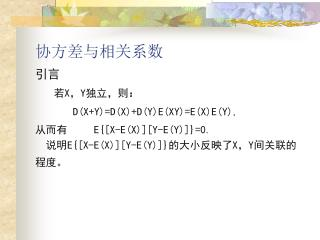DownloadDownload Presentation# 协方差与相关系数

Download Presentation## 协方差与相关系数

- - - - - - - - - - - - - - - - - - - - - - - - - - - E N D - - - - - - - - - - - - - - - - - - - - - - - - - - -
##### Presentation Transcript

1. 协方差与相关系数 引言 若X，Y独立，则： D(X+Y)=D(X)+D(Y)E(XY)=E(X)E(Y), 从而有 E{[X-E(X)][Y-E(Y)]}=0.说明E{[X-E(X)][Y-E(Y)]}的大小反映了X，Y间关联的程度。

2. 三、协方差与相关系数的定义 1．协方差的定义 量E{[X-E(X)][Y-E(Y)]}称为随机变量X与Y的协方差，记为Cov(X，Y)，即 Cov(X，Y)= E{[X-E(X)][Y-E(Y)]}.而当D(X)>0, D(Y)>0时， 称为随机变量X与Y的相关系数。 注释： (1)Cov(X，Y)作为[X-E(X)][Y-E(Y)]的均值，依赖于X，Y的度量单位，选择适当的单位使X，Y的方差是1，协方 差就是相关系数，这能更好的反映X，Y之间的关系，而 不受所用单位的影响。

3. (2)ρXY是一比例常数，并有定义：ρXY=0 X，Y不相关。 (3) ρXY又称为标准协方差。因为设 一般地，数学期望为0，方差为1的随机变量的分布称为标准分布，故ρXY又称为标准协方差。

4. 2．关系公式: (1) 协方差与方差的关系：D(X+Y)=D(X)+D(Y)+2Cov(X，Y) (2) 协方差与数学期望的关系：Cov(X，Y)=E(XY)-E(X)E(Y) 我们常用这个公式计算协方差。 (3)若X，Y独立，则Cov(X，Y)=0,但反之不成立。 3．协方差与相关系数的性质 协方差具有下述性质： (1) Cov(X，Y)= Cov(Y，X)； (2) Cov(aX，bY)= abCov(X，Y)；

5. (3) Cov(X1+X2，Y)= Cov(X1，Y)+ Cov(X2，Y) 相关系数具有下述性质： (1)|ρXY|≦1 ; 证： 由柯西一许瓦兹不等式知 所以 |ρXY|≦1。

6. (2) |ρXY|=1 存在常数a,b使P{Y=aX+b}=1. 意义|ρXY|=1当且仅当Y跟X几乎有线性关系。这在一定程度上说明了相关系数的概率意义。ρXY并不是刻画X，Y之间的“一般”关系，而只是刻画X，Y之间线性相关的程度。 4．计算： (1)用定义求：若X，Y为离散型随机变量 若X，Y为连续型随机变量

7. (2)用公式： 例1 若X、Y的E(X)=-2,E(Y)=4, D(X)=4, D(Y)=9,分别在(1) X、Y相互独立，(2) ρXY=0.5的条件下，求E(Z)=E(3X2-2XY+Y2-3). 解：(1)因为X、Y相互独立，所以E(XY)= E(X) E(Y)； E(Z)=E(3X2-2XY+Y2-3)= 3 E(X2)-2E(X)E(Y)+ E(Y2)-3 =3[D(X)+[E(X)]2]-2E(X)E(Y)+[D(Y)+[E(Y)]2]-3=62；

8. (2) E(Z)= 3[D(X)+[E(X)]2]-2E(XY)+[D(Y)+[E(Y)]2]-3 =24-2[Cov(X,Y)+ E(X)E(Y)]+25-3 =24-2[ρXY+ E(X)E(Y)]+25-3=68. 例2 设=aX+b,=cY+d,(a,c同号),证明：ρ=ρXY。 证：

9. 5．定义若X与Y的相关系数ρXY=0，则称X与Y不相关。5．定义若X与Y的相关系数ρXY=0，则称X与Y不相关。 假设随机变量X，Y的相关系数ρXY存在，当X与Y相互独立时,ρXY=0,即X与Y不相关，反之若X与Y不相关，X与Y却不一定相互独立。 例1: 设(X，Y)在单位圆x2+y2≦1上服从均匀分布，证明：ρXY=0，但X与Y不相互独立。 解: (1)(X，Y)的概率密度为

10. 关于X的边缘密度为 同理，关于Y的边缘密度为

11. 容易看到，(1/2,1/2)是fX(x), fY(y), f(x,y)的 连续点，但 所以X与Y不相互独立。

12. 所以 D(X)=1/4. 同样方法可得 E(Y)=0,D(Y)=1/4. 于是 Cov(X，Y)=E(XY)-E(X)E(Y)=0 所以 ,即 X与Y不相关。

13. 由相关系数性质(2),ρXY并不是刻画X，Y之间的“一般”关系，而只是刻画X，Y之间线性相关的程度。虽然X，Y不相关，但X，Y可以有关系。例如X～U(-1/2,1/2),Y=cosX,则E(X)=0, 因此，ρXY=0，但X，Y有严格的函数关系。那么，是否有特例哪？

14. 例2: 设(X，Y)～N(μ1, μ2 ,σ12, σ22,ρ)，求X与Y的相关系数ρXY 解: X～N(μ1,σ12), E(X)=μ1, D(X)=σ12； Y～N(μ2,σ22)，E(Y)=μ2, D(X)=σ22； 而

15. 所以 ρXY=ρ。

16. 二维正态随机变量的分布完全可由X，Y个别的数学期望、方差以及它们的相关系数所确定。 若(X，Y)服从二维正态分布，那么X和Y相互独立的充要条件为ρ=0，而ρ=ρXY，故知对于二维正态随机变量(X，Y)来说， X与Y不相关与X和Y相互独立是等价的。

17. 小结： 结论1：X与Y相互独立 ρXY=0  X与Y不相关； 反之，ρXY=0 不能推出X与Y相互独立。 结论2：对任意X与Y，以下结论等价ρXY=0  Cov(X，Y)=0  E(XY)=E(X)E(Y) D(X+Y)=D(X)+D(Y)。 结论3：若(X，Y)～N(μ1, μ2 ,σ12, σ22,ρ)，则X与Y相互独立 ρXY=0  X与Y不相关。

18. 四、矩、协方差矩阵的定义 1. 矩的定义1.设X为随机变量，c为任意常数，k为正整数,称量E[(X-c)k]为X关于c点的k阶矩。 比较重要的有两种情况： (1) c=0, 这时，ak=E(Xk)称为X的k阶原点矩； (2) c=E(X), 这时，bk=E[X-E(X)]k称为X的k阶中心矩。 定义2：对正整数k与l，称E(XkYl)为X和Y的k+l阶混合矩；若E{[X-E(X)]k[Y-E(Y)]l}存在，称它为X和Y的k+l 阶混合中心矩。

19. 例1: 设XN(µ,σ2),求:X的k阶中心矩ak(k为正整数)。 解: E(X)=μ, 当k为奇数时ak=0。当k为偶数时，

20. 由此推递关系 而a2=D(x)=σ2,所以当k为偶数时: 所以X的k阶中心矩为 特别地，若XN(0,1),则

21. 1.n维随机变量的协方差矩阵 (1)．二维随机向量的协方差矩阵 二维随机变量(X1,X2)有四个二阶中心矩(设他们存在)，分别记为 写为矩阵的形式： 称为随机变量(X1,X2)的协方差矩阵。

22. 例2: 设(X,Y)N(µ1, µ2,σ12,σ22,),求向量(X，Y)＇的均值μ与协方差矩阵。 解: E(X)=μ1，E(Y)=μ2， 所以(X，Y)的均值为μ=(μ1,μ2)协方差矩阵为

23. (2)推广 对于n维随机向量(X1,X2,…,Xn),把向量(X1,X2,…,Xn)用列向量形式表示并记为X，即X=(X1,X2,…,Xn)。 定义 设X=(X1,X2,…,Xn)为n维随机向量,并记μi=E(Xi)， 则称μ=(μ1,μ2,…,μn)为向量X的数字期望或均值，称矩阵 为向量X的协方差矩阵。

24. 3.矩、协方差矩阵的性质 协方差矩阵具有以下性质： (1)协方差矩阵对角线上的元素Cii为Xi的方差即Cii=D(Xi) i=1，2，…，n； (2)协方差矩阵C为对称矩阵,即Cij=Cji ,i，j=1，2，…，n； (3)C为非负定矩阵,即对于任意实向量t=(t1，t2，…，tn),有tCt≥0； 证：性质(1)，(2)显然，只证(3)

25. 4．多维正态分布及其性质 二维正态随机向量X=(X1，X2)的概率密度为 引入下面记号

26. 经简单的运算可得出 于是X=(X1，X2)的概率密度可写成

27. 并且,若将二维正态分布密度用向量和矩阵写成上式，那么上式中的向量μ=(μ1，μ2)正是X的均值，矩阵C正是X的协方差阵，而且当|ρ|<1时C为正定矩阵。 上式推广至n维正态分布的情况，于是有以下定义： (1)定义 若n维随机向量X=(X1，…，Xn)的概率密度为 其中X=(X1，…，Xn),μ=(μ1，μ2，…，μn) 为n维实向量，C为n阶正定对称矩阵，则称向量 X=(X1，…，Xn)服从n维正态分布，记为X～N(µ,C) . 对于n维正态分布X～N(µ,C) ，X的期望为µ，X的协方差矩阵为C。

28. (2) 性质 n维正态分布具有下述性质： (1)n维随机向量(X1，…，Xn)服从n维正态分布的充要条件是X1，…，Xn的任意线性组合l1X1+l2X2+…+lnXn(l1,l2,…,ln不全为0)服从一维正态分布。 (2)若X=(X1,…,Xn)～N(µ,C)，设Y=(Y1,Y2,…,Ym)=AX,即Yi为Xj(j=1，2，…，n)的线性函数,i=1，2，…，m，则Y～N(Aµ，ACA)，其中A为－m行n列且秩为m的矩阵。 (3)设(X1，…，Xn)服从n维正态分布，则“X1，…，Xn”相互独立与“X1，…，Xn两两不相关”是等价的。

29. 例3:设X～N（0，1），Y～N(0，1 ),若X与Y相互独立，求E(|X-Y|)。 解: 令Z=X-Y，问题化为求E(|Z|)，为求E(|Z|),我们先求出Z的分布密度. 由于(X，Y)服从二维正态分布，由性质知Z服从一维正态分布，而E(Z)=E(X-Y)=E(X)-E(Y)=0，D(Z)=D(X-Y)=D(X)+D(Y)=2，故Z～N（0，2），即Z的分布密度为 于是

30. 例4: 设,问X与Z是 否独立? 解: 由于 由性质知(X，Z)服从二维正态分布，再由性质知判断X与Z是否独立等价于判断X与Z是否不相关。 D(X)=32， D(Y)=42，ρXY=-1/2,

31. 于是ρXY=0 所以X与Z不相关，由此可得X与Z相互独立。 小结： 1． 2.由于正态分布在概率论中有其特殊地位，因此 对多维正态分布的性质及其应用要较好地掌握。